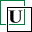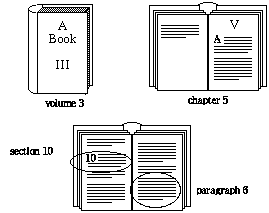Tumbler Arithmetic

Imagine a row of books on a shelf numbered 1, 2, 3, ...Chapters in each book could be similarly numbered, so that Chapter Five in Volume Three would be represented as 3.5. This could be extended arbitrarily, so that 3.5.10.6 represents Volume Three, Chapter Five, Section Ten, Paragraph Six.If you had Volume Three open to the place mentioned above, and were told to move forward two volumes, sixteen chapters, and three sections, you would doubtless put Volume Three back, take down Volume Five, then count forward sixteen chapters and three sections. This may be represented as a non-commutative arithmetic operation:

3. 5.10.6 + 2.16. 3  5.16. 3 This arithmetic gives different roles to the two numbers: the first specifies where something is, the second specifies an offset, a distance to move forward. Only the first digit of the original location matters, because in counting ahead by volumes, the initial paragraph, section, and chapter make no difference.

A similar case, but with an offset remaining within the first volume, is shown in this example: 25.6.46.93 + 0.0. 3. 1.21 25.6.49. 1.21 The following rule describes this sort of offset procedure on this sort of numbering system: * Evaluating from the most to the least significant entries, the elements of the final position are equal to the corresponding elements of the initial position as long as the corresponding elements of the offset are zero.

* The first non-zero offset element is added to the corresponding element of the initial position.

* The remaining elements of the final position are equal to the remaining elements of the offset.
The numbers in this system are called tumblers, and this addition property is the foundation of tumbler arithmetic.

This sort of numbering system is widely used in military documents, to permit insertion of material at any point without renumbering subsequent parts. Note that if one does not identify particular parts of a tumbler with any particular level of text, then one can insert a book's worth of material (with appropriately numbered chapters, etc.) between any two characters. This merely involves appending to the number for the first character a "." followed by a "1" (for the first item inserted between those two characters). The body of the book could be numbered by appending further tumbler elements.